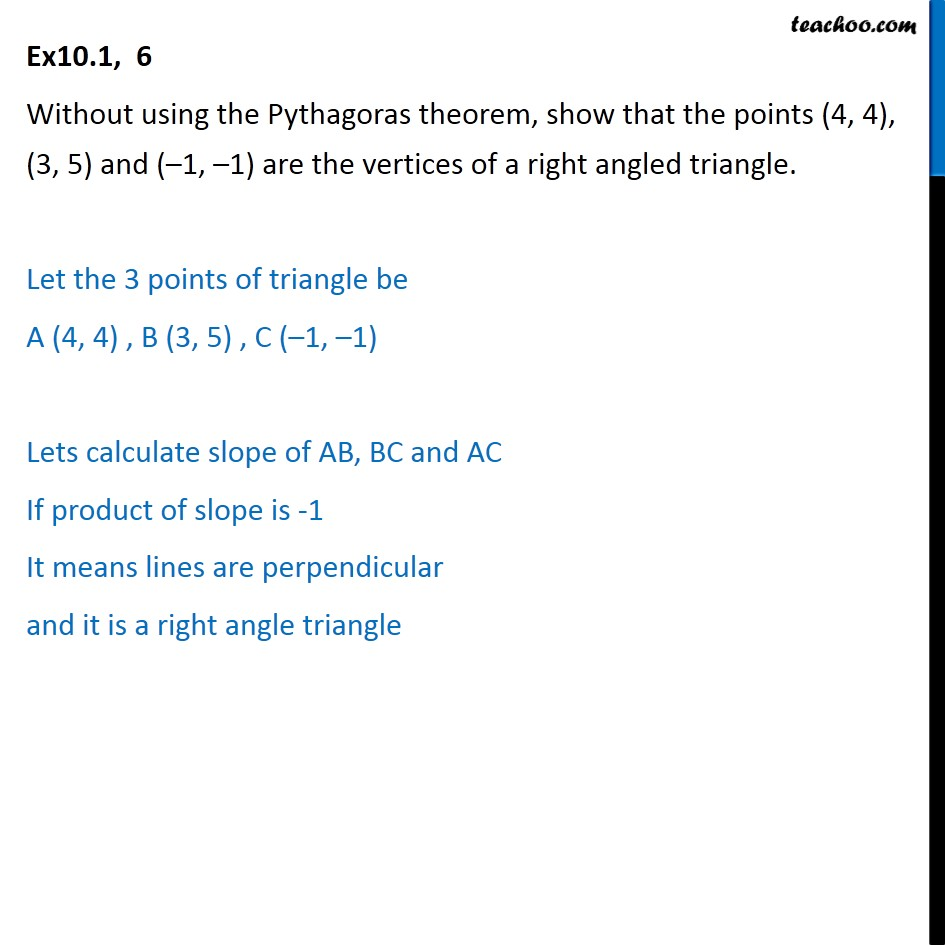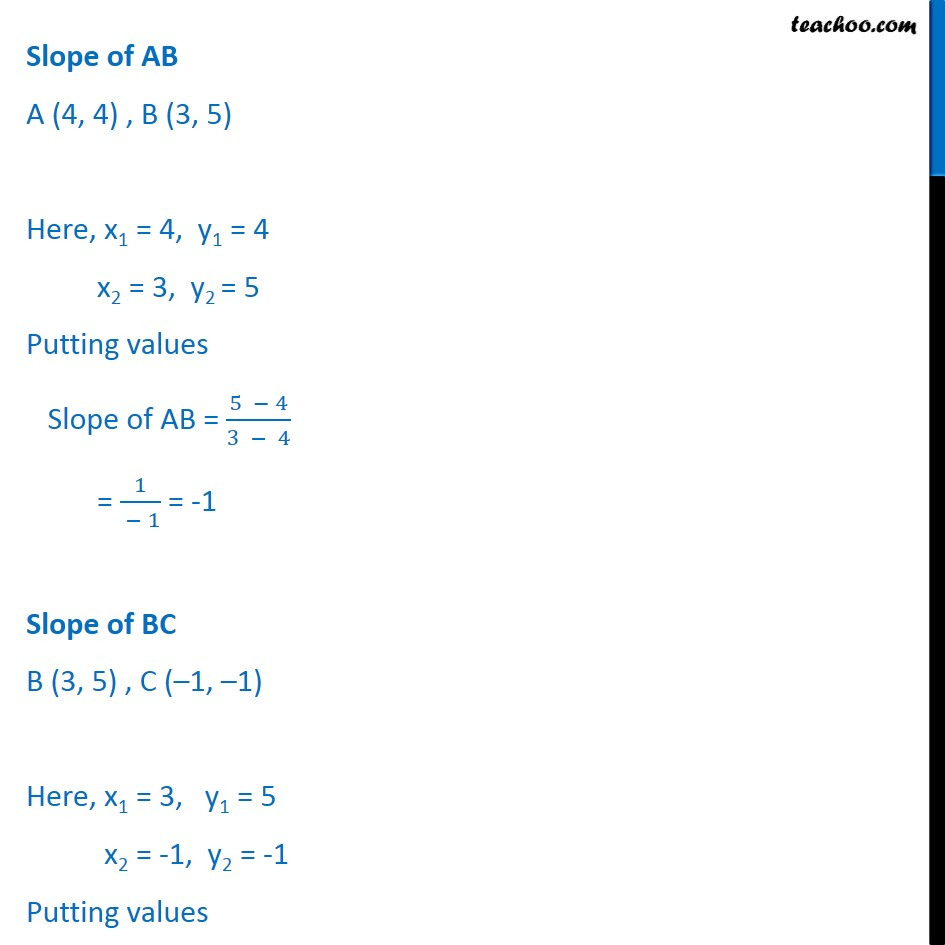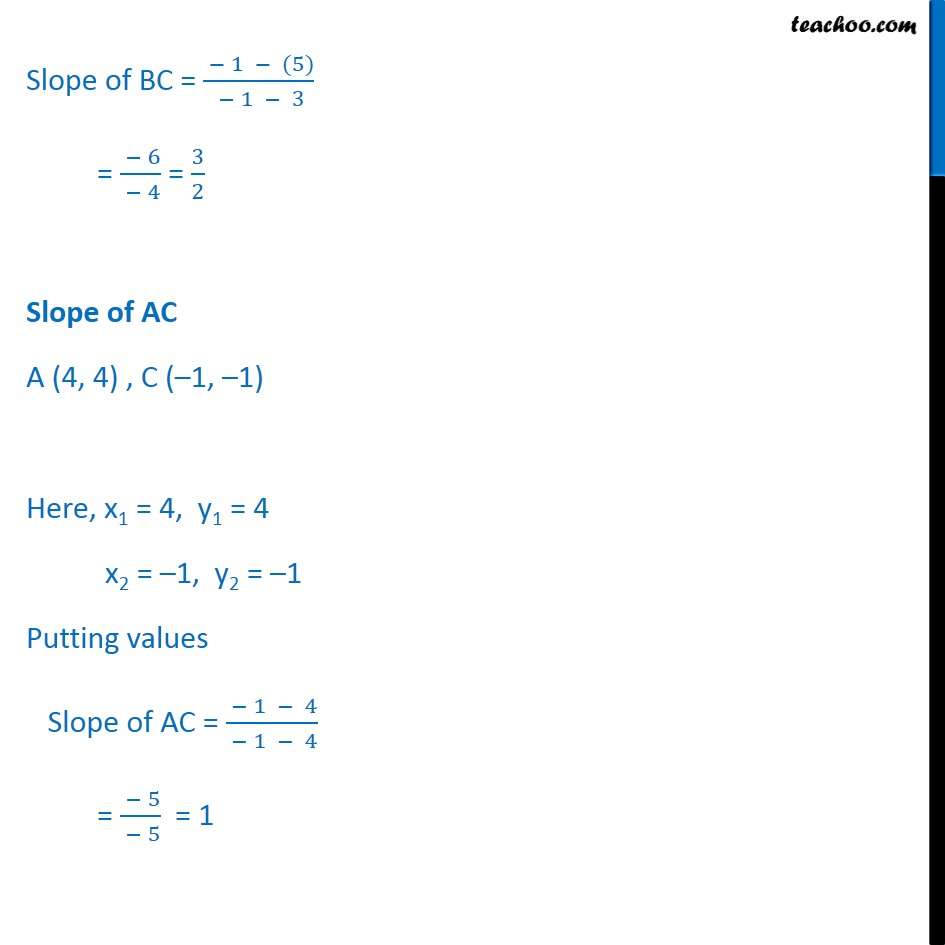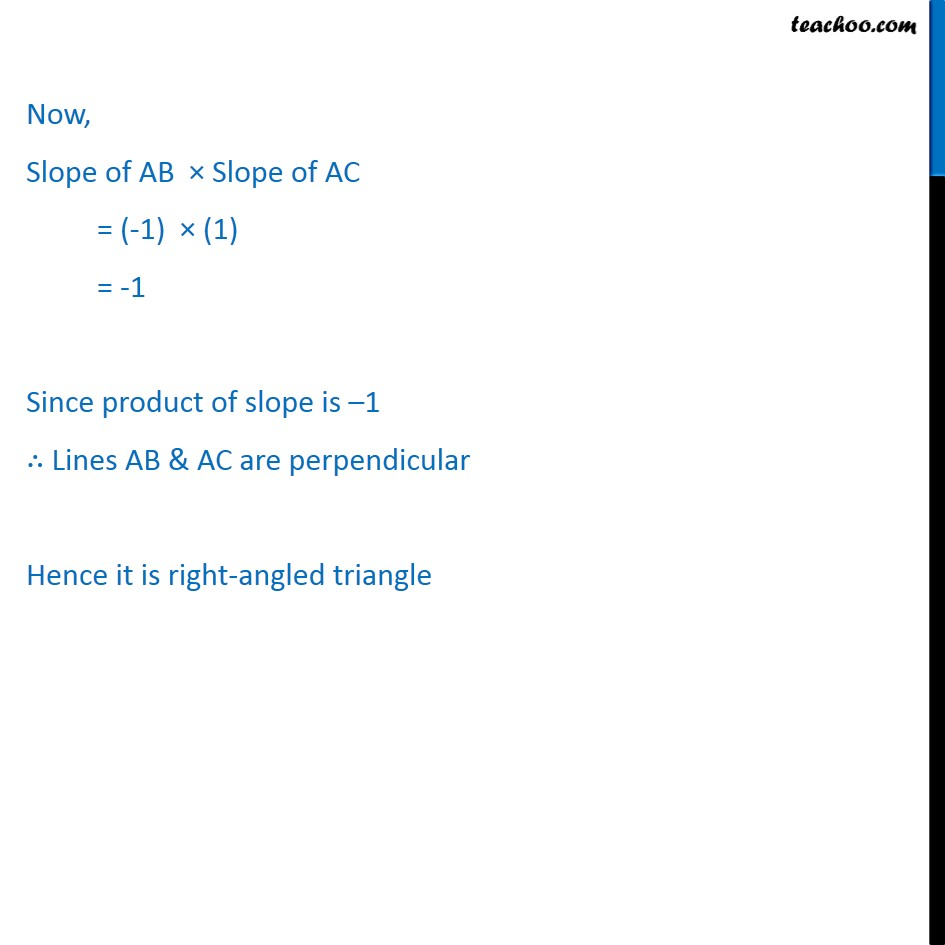Ex 9.1

Chapter 9 Class 11 Straight Lines
Serial order wiseLearn in your speed, with individual attention - Teachoo Maths 1-on-1 Class

### Transcript

Ex10.1, 6 Without using the Pythagoras theorem, show that the points (4, 4), (3, 5) and (–1, –1) are the vertices of a right angled triangle. Let the 3 points of triangle be A (4, 4) , B (3, 5) , C (–1, –1) Lets calculate slope of AB, BC and AC If product of slope is -1 It means lines are perpendicular and it is a right angle triangle Slope of AB A (4, 4) , B (3, 5) Here, x1 = 4, y1 = 4 x2 = 3, y2 = 5 Putting values Slope of AB = (5 − 4)/(3 − 4) = 1/( − 1) = -1 Slope of BC B (3, 5) , C (–1, –1) Here, x1 = 3, y1 = 5 x2 = -1, y2 = -1 Putting values Slope of BC = ( − 1 − (5))/( − 1 − 3) = ( − 6)/( − 4) = 3/2 Slope of AC A (4, 4) , C (–1, –1) Here, x1 = 4, y1 = 4 x2 = –1, y2 = –1 Putting values Slope of AC = ( − 1 − 4)/( − 1 − 4) = ( − 5)/( − 5) = 1 Now, Slope of AB × Slope of AC = (-1) × (1) = -1 Since product of slope is –1 ∴ Lines AB & AC are perpendicular Hence it is right-angled triangle